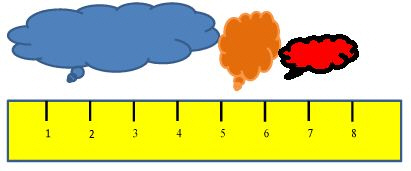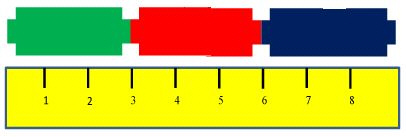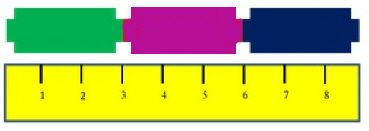Name:    1MD2.1

 CompletionComplete each statement. 1. How many inches long are the green and red shapes togehter in the picture? (Put ONLY a number.  NO WORDS.)2. About how many inches long are the orange and red clouds together in the picture?3. About how many inches long are the blue and orange clouds together in the picture?4. How many inches long is the shortest cloud?5. How many inches long are the blue and green shapes together in the picture?6. How many inches long are the purple and green shapes together in the picture?7. How many inches longer is the red shape than the purple one in the picture?8. How many inches shorter is the purple shape than the green one in the picture?9. How many inches longer is the red shape than the green one in the picture?10.How much longer is the blue cloud than the red cloud in the picture?